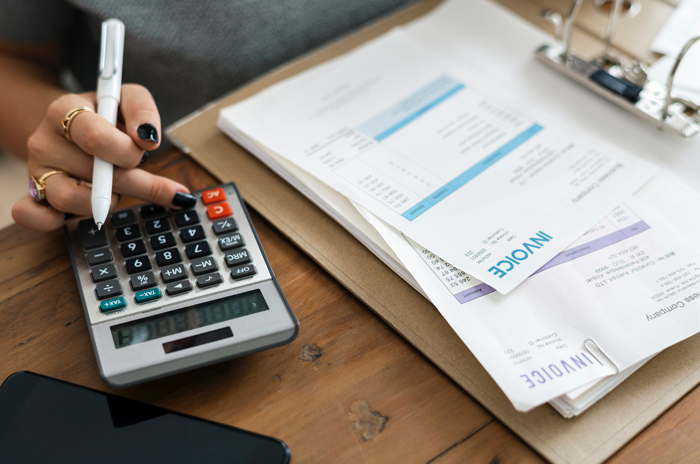# How to Calculate Your Monthly Interest

0
16The supplier or lender of funds normally wants to earn an income and the user or borrower will generally be prepared to pay for the right to use the accumulated funds. This is known as an interest rate. Whether that may be money loaned from the bank for vehicle finance or a home loan.

An interest rate is that added fee to your outstanding debt. An interest rate applies to the total unpaid portion of your loan or credit card balance. Therefore it’s critical to know what your interest rate is or your outstanding debt will increase even though you’re making payments.

The interest rate is calculated by dividing the amount of interest by the principal. The interest rate charged on the principal is dependent on the individuals risk profile. Lower interest is charged on lower risk and higher interest is charged on higher risk. Interest rates often change as a result of inflation, Reserve Bank policies, and supply of or demand for credit.

Generally, interest rates for access to credit cards, mortgage or loans are charged on an annual basis, known as the Annual Percentage Rate (APR). Conversely, when consumers earn interest for savings or investments the interest rate is expressed as an Annual Percentage Yield (APY).

Interest rates refer to the cost of borrowing money. When a bank lends you money, they charge you a percentage of that loan, which is payable monthly.

This percentage is the interest rate a term that represents the individual rates charged in the lending and borrowing process. There are various types of interest rates that exist, all of which are expressed as an APR of the total amount borrowed.

Various banks like Nedbank, FNB, and Old Mutual provide various online calculators for you to calculate your interest rate. Whether if it’s the interest rate on your savings account. To help you understand the interest you can earn or calculate the interest earned for a chosen amount through a fixed Deposit interest calculator.